# The GLM Procedure

### Computational Resources

Subsections:

#### Memory

For large problems, most of the memory resources are required for holding thematrix of the sums and crossproducts. The section Parameterization of PROC GLM Models describes how columns of thematrix are allocated for various types of effects. For each level that occurs in the data for a combination of classification variables in a given effect, a row and a column forare needed.

The following example illustrates the calculation. Suppose A has 20 levels, B has 4 levels, and C has 3 levels. Then consider the model

proc glm;
class A B C;
model Y1 Y2 Y3=A B A*B C A*C B*C A*B*C X1 X2;
run;


Thematrix (bordered by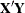and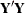) can have as many as 425 rows and columns: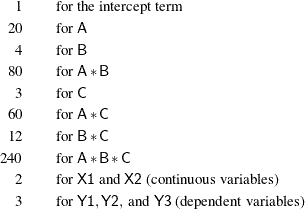The matrix has 425 rows and columns only if all combinations of levels occur for each effect in the model. For m rows and columns,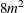bytes are needed for crossproducts. In this case,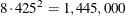bytes, or about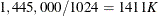.

The required memory grows as the square of the number of columns of; most of the memory is for the A*B*C interaction. Without A*B*C, you have 185 columns and need 268K for. Without either A*B*C or A*B, you need 86K. If A is recoded to have 10 levels, then the full model has only 220 columns and requires 378K.

The second time that a large amount of memory is needed is when Type III, Type IV, or contrast sums of squares are being calculated. This memory requirement is a function of the number of degrees of freedom of the model being analyzed and the maximum degrees of freedom for any single source. Let Rank equal the sum of the model degrees of freedom, MaxDF be the maximum number of degrees of freedom for any single source, and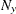be the number of dependent variables in the model. Then the memory requirement in bytes is 8 times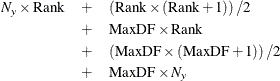The first two components of this formula are for the estimable model coefficients and their variance; the rest correspond to,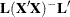, and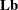in the computation of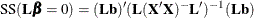. If the operating system enables SAS to run parallel computational threads on multiple CPUs, then GLM will attempt to allocate another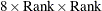bytes in order to perform these calculations in parallel. If this much memory is not available, then the estimability calculations are performed in a single thread.

Unfortunately, these quantities are not available when thematrix is being constructed, so PROC GLM might occasionally request additional memory even after you have increased the memory allocation available to the program. If you have a large model that exceeds the memory capacity of your computer, these are your options:

• Eliminate terms, especially high-level interactions.

• Reduce the number of levels for variables with many levels.

• Use the ABSORB statement for parts of the model that are large.

• Use the REPEATED statement for repeated measures variables.

• Use PROC ANOVA or PROC REG rather than PROC GLM, if your design allows.

A related limitation is that for any model effect involving classification variables (interactions as well as main effects), the number of levels cannot exceed 32,767. This is because GLM internally indexes effect levels with signed short (16-bit) integers, for which the maximum value is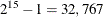.

#### CPU Time

Typically, if the GLM procedure requires a lot of CPU time, it will be for one of several reasons. Suppose that the input data has n rows (observations) and the model has E effects that together produce a design matrixwith m columns. Then if m or n is relatively large, the procedure might spend a lot of time in any of the following areas:

• collecting the sums of squares and crossproducts

• solving the normal equations

• computing the Type III tests

The time required for collecting sums and crossproducts is difficult to calculate because it is a complicated function of the model. The worst case occurs if all columns are continuous variables, involving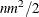multiplications and additions. If the columns are levels of a classification, then only m sums might be needed, but a significant amount of time might be spent in look-up operations. Solving the normal equations requires time for approximately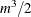multiplications and additions, and the number of operations required to compute the Type III tests is also proportional to both E and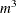.

Suppose that you know that Type IV sums of squares are appropriate for the model you are analyzing (for example, if your design has no missing cells). You can specify the SS4 option in your MODEL statement, which saves CPU time by requesting the Type IV sums of squares instead of the more computationally burdensome Type III sums of squares. This proves especially useful if you have a factor in your model that has many levels and is involved in several interactions.

If the operating system enables SAS to run parallel computational threads on multiple CPUs, then both the solution of the normal equations and the computation of Type III tests can take advantage of this to reduce the computational time for large models. In solving the normal equations, the fundamental row sweep operations (Goodnight; 1979) are performed in parallel. In computing the Type III tests, both the orthogonalization for the estimable functions and the sums of squares calculation have been parallelized (if there is sufficient memory).

The reduction in computational time due to parallel processing depends on the size of the model, the number of processors, and the parallel architecture of the operating system. If the model is large enough that the overwhelming proportion of CPU time for the procedure is accounted for in solving the normal equations and/or computing the Type III tests, then you can expect a reduction in computational time approximately inversely proportional to the number of CPUs. However, as you increase the number of processors, the efficiency of this scaling can be reduced by several effects. One mitigating factor is a purely mathematical one known as "Amdahl’s law," which is related to the fact that only part of the processing time for the procedure can be parallelized. Even taking Amdahl’s law into account, the parallelization efficiency can be reduced by cache effects related to how fast the multiple processors can access memory. See Cohen (2002) for a discussion of these issues. For additional information about parallel processing in SAS, see the chapter on "Support for Parallel Processing" in SAS Language Reference: Concepts.